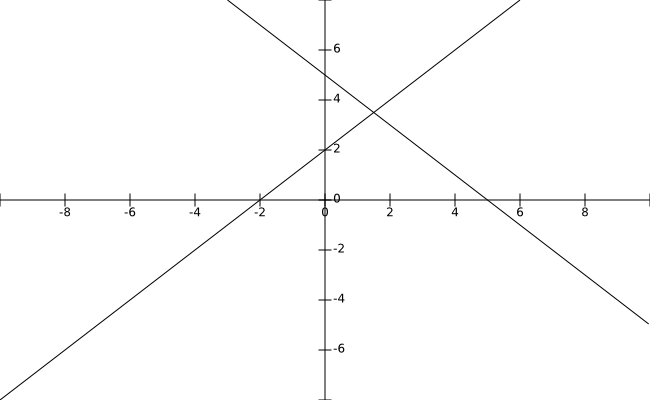Question 63

# Let g(x) = max(5 - x, x + 2). The smallest possible value of g(x) is

Solution

Smallest possible value would be at 5-x = x+2 i.e. x= 1.5 as shownSubstituting we get smallest value as 3.5.